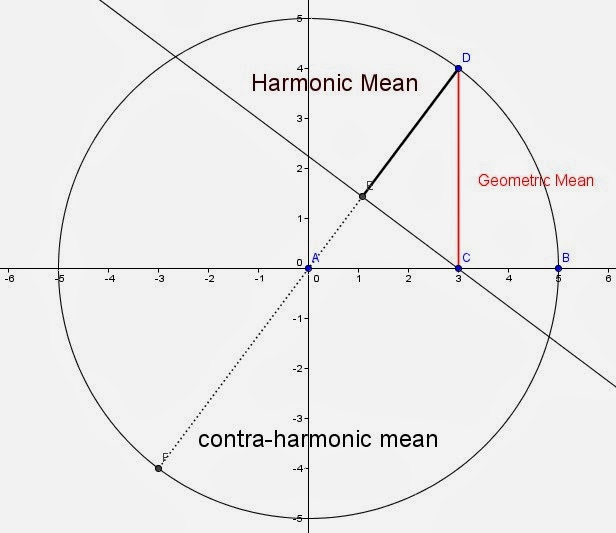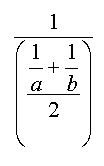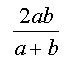## Wednesday 4 December 2019

### The Harmony of the Harmonic Mean, and more Related Problems

Things happen in threes according to the old myth, and in this case it was true. I was doing some research on the early history of a mathematical problem often called the "cistern" problem. You probably know the type; "If one pipe can fill a cistern in 6 hours and another can fill it in four hours, how long would it take both pipes working together." While I was working on that, I got a nice article sent to me on the first proof that the harmonic sequence diverges... and then, I was reading a blog by Dave Marain Math Notationsin which he posed a problem that asked, in its general form, given a square inscribed in a right triangle (with one corner at the right angle of the triangle), what is the length of a side of the square in terms of the legs of the triangle.

So what do all these have in common with each other. dare I say what makes them in "harmony"?.... the answer is Harmony, or at least the mathematical relationship of the harmonic mean.

To the early Greeks, if Nichomachus can be believed, all the means were descriptive of musical relations. Much is often made of the Harmonic Mean in relation to a musical sense, but this may not represent the Greek view. Euclid used the word enarmozein to describe a segment that just fits in a given circle. The word is a form of the word Harmozein which the more competent Greek Scholars tell me means to join or to fit together. Jeff Miller's Web site on the first use of Mathematical terms contains a reference to the very early origin of the harmonic mean, 'A surviving fragment of the work of Archytas of Tarentum (ca. 350 BC) states, 'There are three means in music: one is the arithmetic, the second is the geometric, and the third is the subcontrary, which they call harmonic.' The term harmonic mean was also used by Aristotle. "

The harmonic mean is one of the classic old Greek means called Pythagorean means because they were, supposedly, known to the Pythagoras or his followers. The harmonic mean is associated with the musical interval known as the Perfect fourth. An octave change upwards in pitch reflects a doubling of the wavelength frequency (a 1:2 ratio), the harmonic mean of 1 and 2 is 4/3.

The actual definition of the harmonic mean usually sounds convoluted to students. The harmonic mean is the reciprocal of the average of the reciprocals of the values. The algebraic notation is only a little clearer. For a set of numbers xi the harmonic mean, H, is given by $H = \frac{n}{ \frac{1}{x_1} + \frac {1}{x_2} + \dotsb+ \frac {1}{x_n} }$

Another classic geometry illustration  is used to show the relationship between the means of two values in a circle.  When the diameter is divided into two parts, a and b, then the relation between the Arithmetic mean which equals the length of the radius, the Geometric mean and Harmonic mean will always be related by  $A \ge G \ge H$

In the trapezoidal view of the harmonic mean of two numbers, the arithmetic mean (green) joins the midpoints of the two non-parallel sides and the contra-harmonic mean (blue) is the same distance away from the arithmetic mean as the harmonic mean.A slightly different version shows the contra -harmonic mean which is equal to the length of the diameter less the harmonic mean.    The contr-harmonic mean is the ratio of the sum of the squares divided by the sum of the values, or $\frac{x_1^2 + x_2^2 + \dotsb + x_n^2}{x_1 + x_2 + \dotsb + x_n}$

My search for the early roots of the cistern problem had taken me back to Heron's Metre'seis around the year fifty of the common era. The problem became a staple in arithmetics and problem books and was used by Alcuin (775) and appears in the Lilavati of Bhaskara (1150). I found the illustration I used on the blog for The First Illustrated Arithmetic a few days ago, from the 1492 arithmetic, Trattato di aritmetica by Filippo Calandri.

The solution to a cistern problem is the harmonic mean of the times taken by each pipe. For example, one problem asks "If one pipe can fill a cistern in three hours, and a second can fill it in five hours, how fast will the two pipes take to fill the cistern if both are opened at once. The solution is given by finding the average rate of fill of the two rates, the harmonic mean of three and five, which is three and three-quarter hours. But as the name "mean" suggest, that's the average rate of the two so working together, they would take one-half the time, one and seven-eighths hours, or about an hour and 53 minutes.

The Harmonic mean is the reciprocal of the mean of the reciprocals of the values, so for values a and b, the harmonic mean is given bywhich for two numbers can be simplified to the more economicalHeron might have been the first recorded example of a cistern problem, but a problem calling on the reader to use the harmonic mean occurs even earlier in the Rhind Mathematical Papyrus, now located in the British Museum, in problem 76. The problem involves making loaves of bread with different qualities, but the solution is still the harmonic mean. (I have learned from David Singmaster's Chronology of Recreational Mathematics that the cistern problem appeared, perhaps 300 years before Heron's use, in China by Chiu Chang Suan Shu (around 150 BC).

The series of terms formed by the reciprocals of the positive integers is a common torment for college students in their first introduction to analysis. The sequencein which each number gets smaller and smaller seems to very slowly approach some upper limit. Even after adding 250,000,000 terms, the sum is still less than twenty, and yet... in the mid 1300's, Nichole d'Oresme showed that it will eventually pass any value you can name. In short, it diverges, slowly, very, very slowly, to infinity. Even when warned, it seems like students want to believe it converges. A well-known anecdote about a teacher trying to get student's to remember that it diverges goes:
"Today I said to the calculus students, “I know, you’re looking at this series and you don’t see what I’m warning you about. You look and it and you think, ‘I trust this series. I would take candy from this series. I would get in a car with this series.’ But I’m going to warn you, this series is out to get you. Always remember: The harmonic series diverges. Never forget it.”<\blockquote>

By the way, each number in the harmonic series is the harmonic mean of the numbers on each side of it (so 1/2 and 1/4 have a harmonic mean of 1/3), and in fact, of any numbers equally spaced away from it such as 1 and 1/5 also have a harmonic mean of 1/3.

And then, I came across that little problem of a square inscribed in a right triangle. If the two legs are a and b, then the sides of the square will have a length equal to the one-half the harmonic mean of a and b .  More generally, a square inscribe in any triangle with one side along a base will have sides equal to one half the harmonic mean of the base and the altitude to that base.  There are lots of other interesting problems that yeild to the use of the harmonic mean, and I mean to write again on that collection.

So I guess things do come in threes, unless I come across another one, but whether it comes in threes or fours, it all seems to work together, in perfect harmony.

Many students who struggle with a different puzzle type problem might want to investigate how it too, relates to the harmonic mean, the one where they ask, If you drive to grandmother's house at 60 miles per hour and drive home at 40 miles per hour, what was your average speed for the round trip?

Here are a few other variations, and the only hint you get is ....... well, look at the title of the blog:

The problem above is often called the crossed ladders problem, as it is on the Wikipedia site where I found this image. The idea is that the two ladders are each resting against the foot of a building and leaning against the opposite building. Given the heights A and B at which they touch the opposite buildings, the challenge is to find the height, h at which they cross.  It appeared  at least by 850 AD in a text by Mahavira, an Indian Mathematician.

Here is the "square inscribed in a right triangle" from above whose legs are A units and B units (with one corner of the square at the right angle of the triangle), what is the length of a side of the square?
A 3-d version of the same problem may be more of a challenge for the more talented students:

A cube inscribed in a right rectangular tetrahedron with legs of a, b, and c has a cube inscribed with one vertex at (0,0,0).

What is the length of an edge of the cube?

Another with a triangle,

Given a 5-12-13 Right Triangle, Find a relation between the radius of the inscribed circle and the altitudes.   Is this property true of a non-right triangle?

For the student of the conics, In the ellipse $9x^2+4y^2=36$, find the length of the semi-latus rectum.

Addendum: Recently came across this neat one from Antonio Gutierrez@gogeometry.  Note the radii of the two Archimedean Twins.

There are dozens more, so just to extend the collection, send your favorite problem related to the harmonic mean, and I'll update as they come along.